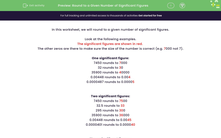# Round to a Given Number of Significant Figures

In this worksheet, students will practise rounding numbers to a given amount of significant figures.Key stage:  KS 3

Curriculum topic:   Number

Curriculum subtopic:   Round/Approximate Numbers Appropriately

Difficulty level:#### Worksheet Overview

In this worksheet, we will round to a given number of significant figures.

Look at the following examples.

The significant figures are shown in red.

The other zeros are there to make sure the size of the number is correct (e.g. 7000 not 7).

One significant figure:

7450 rounds to 7000

32 rounds to 30

35900 rounds to 40000

0.00448 rounds to 0.004

0.0000487 rounds to 0.00005

Two significant figures:

7450 rounds to 7500

32.5 rounds to 33

295 rounds to 300

35900 rounds to 36000

0.00448 rounds to 0.0045

0.0000401 rounds to 0.000040

Three significant figures:

7450 rounds to 7450

32.99 rounds to 33.0

35910 rounds to 35900

35990 rounds to 36000

0.004487 rounds to 0.00449

0.00004895 rounds to 0.0000490

Now let's consolidate your understanding by attacking some questions.

Over to you.### What is EdPlace?

We're your National Curriculum aligned online education content provider helping each child succeed in English, maths and science from year 1 to GCSE. With an EdPlace account you’ll be able to track and measure progress, helping each child achieve their best. We build confidence and attainment by personalising each child’s learning at a level that suits them.

Get started